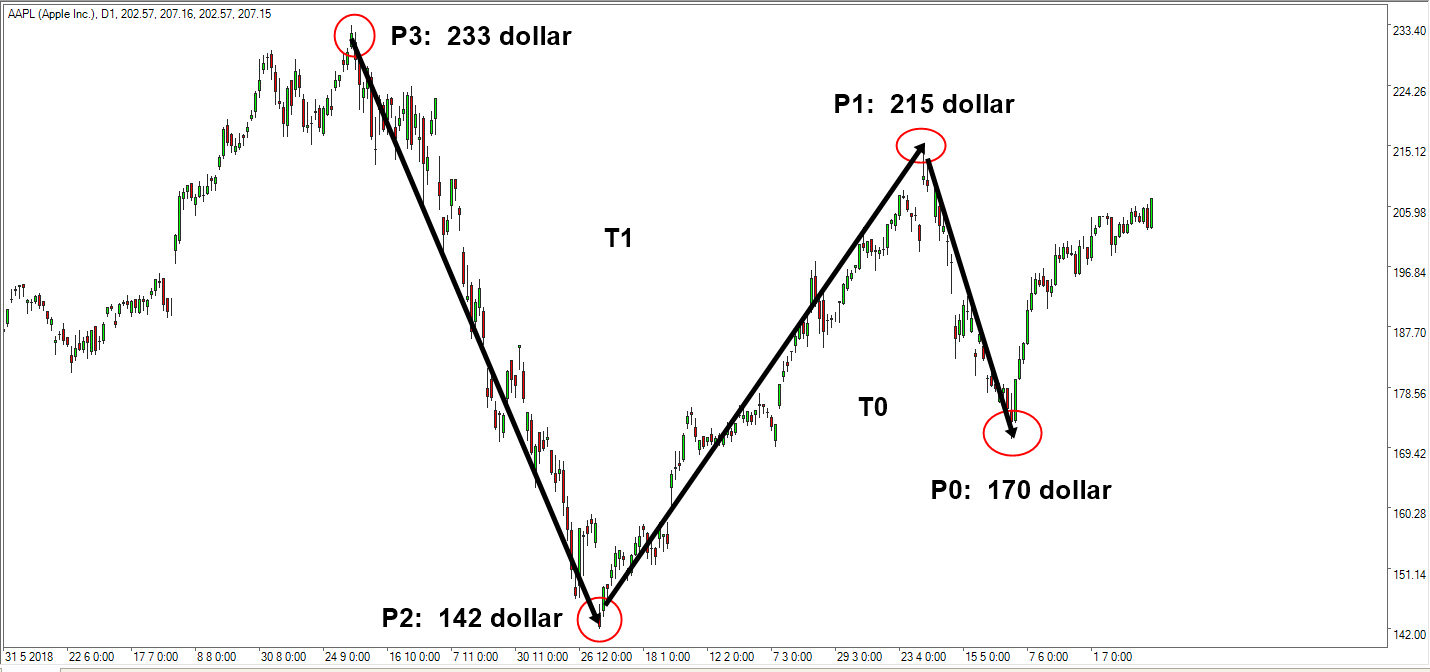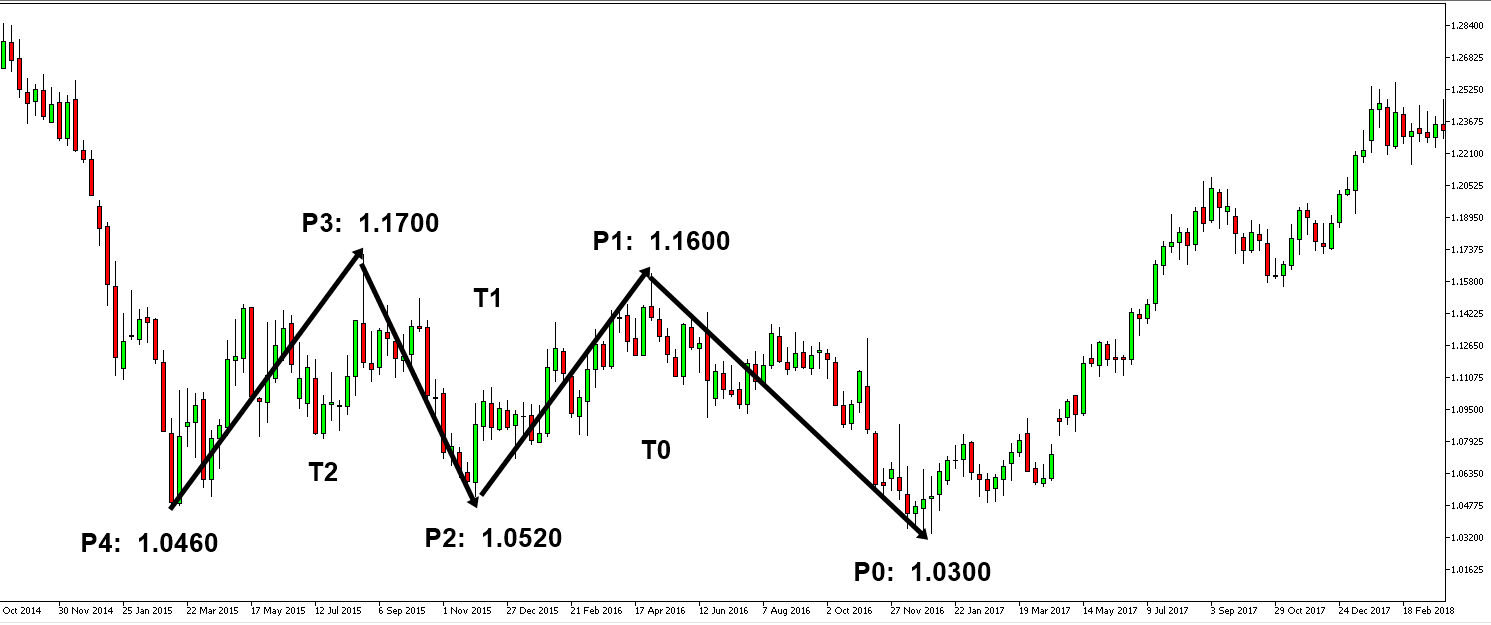Questions on Defining Profitable Patterns

1. Find wrong statement
a) Retracement ratio (R) is calculated by dividing right swing by left swing of a triangle.
b) Expansion ratio (E) is calculated by dividing right swing by left swing of a triangle.
c) Closing Retracement ratio (C) is calculated by dividing right swing by left swing of a triangle.
d) Factored Expansion (F) is calculated by dividing right swing of the first triangle by left swing of the first triangle.

2. Below is Apple’s Stock price. Calculate height of right swing (Y0) and left swing (Y1) of Triangle 0 (T0)3. Below is Apple’s Stock price. Calculate height of right swing (Y1) and left swing (Y2) of Triangle 1 (T1)4. Below is Apple’s Stock price. Calculate Retracement ratio (R0) at P0 for Apple’s Stock price5. Below is Apple’s Stock price. Calculate Retracement ratio (R1) at P0 for Apple’s Stock price6. Below is Apple’s Stock price. Calculate Expansion ratio (E0) at P0 for Apple’s Stock price7. Below is EURUSD price. Calculate height of right swing (Y0) and left swing (Y1) of Triangle 0 (T0)8. Below is EURUSD price. Calculate height of right swing (Y1) and left swing (Y2) of Triangle 1 (T1)9. Below is EURUSD price. Calculate height of right swing (Y2) and left swing (Y3) of Triangle 2 (T2)10. Below is EURUSD price. Calculate Retracement ratio (R0) at P0 for EURUSD price11. Below is EURUSD price. Calculate Retracement ratio (R1) at P0 for EURUSD price12. Below is EURUSD price. Calculate Retracement ratio (R2) at P0 for EURUSD price13. Below is EURUSD price. Calculate Closing Retracement (C0) for T0, T1 and T2.14. Below is EURUSD price. Calculate Expansion ratio at P0.15. Below is EURUSD price. Calculate Factored Expansion ratio for T0: 1 by T2: 1Below is the landing page for Harmonic Pattern Plus, Harmonic Pattern Scenario Planner and X3 Chart Pattern Scanner in MetaTrader. All these products are also available from www.mql5.com too.